## Overview

Tiramisu is a polyhedral compiler for dense and sparse deep learning and data parallel algorithms. It provides a simple C++ API for expressing algorithms and how these algorithms should be optimized by the compiler.

The Tiramisu compiler is based on the polyhedral model thus it can express a large set of loop optimizations and data layout transformations. Currently it targets (1) multicore X86 CPUs, (2) Nvidia GPUs, (3) Xilinx FPGAs (Vivado HLS) and (4) distributed machines (using MPI). It is designed to enable easy integration of code generators for new architectures.

## Where to Use Tiramisu?Image Processing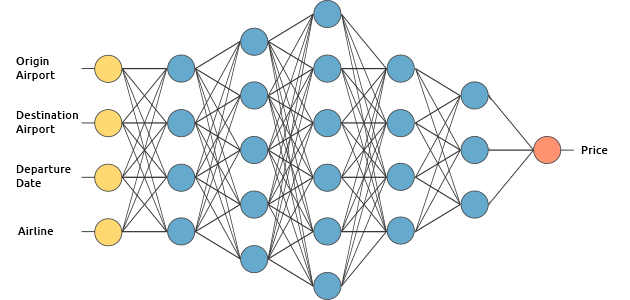Deep LearningScientific Computing

## Why Tiramisu?

• Tiramisu is the only open source DNN compiler that optimizes sparse DNNs.
• Tiramisu supports optimizing RNNs.
• Tiramisu can target distributed architectures (e.g., Cerebras DNN accelerator, distributed systems, …).
• Tiramisu is a polyhedral compiler, therefore:
• It can perform complex loop transformations (such as skewing for RNN optimizaiton).
• It can express programs with cycles in their data-flow graph (e.g., RNNs).
• It supports naturally non-rectangular iteration spaces.
• It uses dependence analysis to guarantee the correctness of optimizations.

The following post provides a more detailed comparison between Tiramisu, Halide and TVM.

## Performance in Deep Learning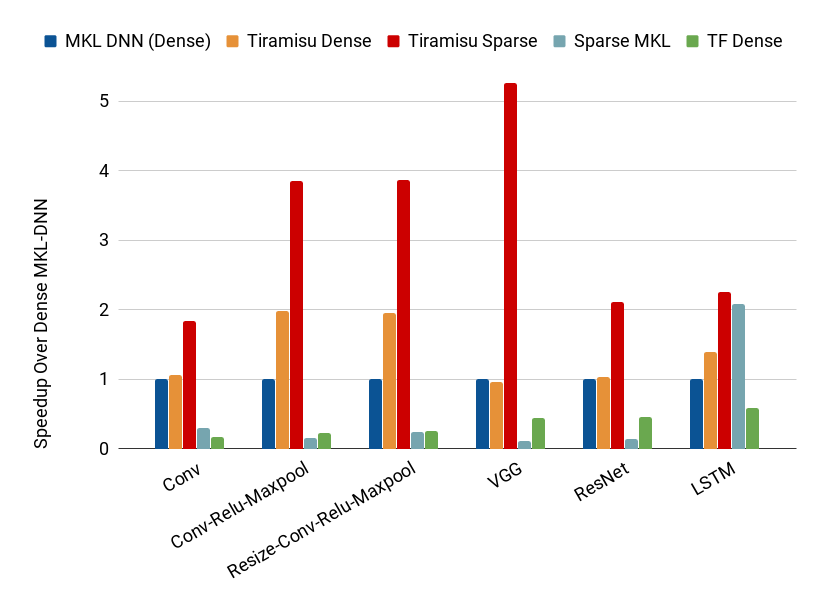Comparison between Tiramisu (dense and sparse), MKL-DNN (dense) and sparse MKL on multi-core CPU.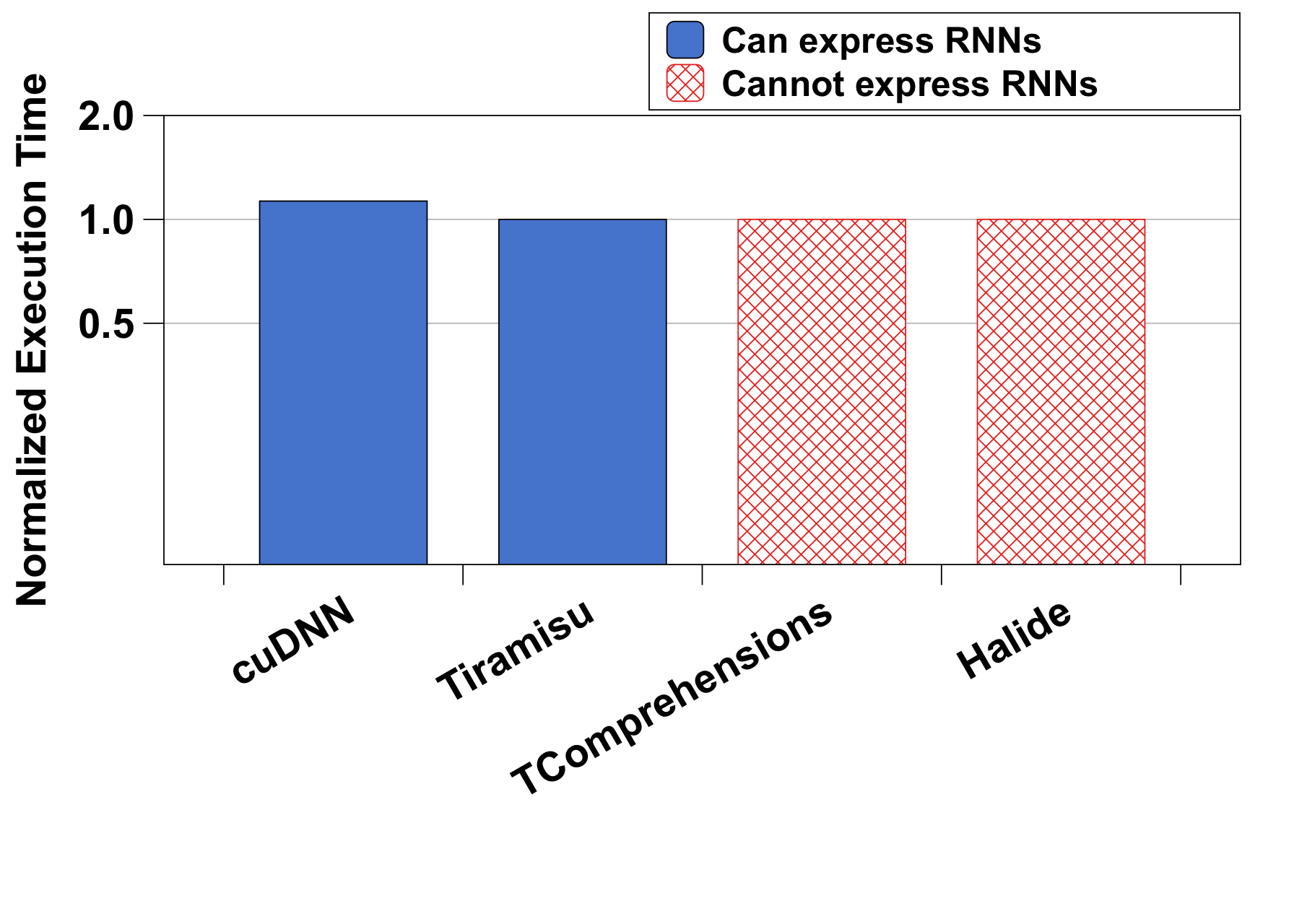Performance of LSTM implemented in Tiramisu compared to cuDNN (GPU) (**).

(*) Standard DNN data sizes are used. The density level (non-zero elements) is 20% for all the benchmarks except VGG where we use 2% (the density levels are obtained from state-of-the-art weight compression techniques.

(**) Tensor Comprehensions and Halide cannot express LSTM because LSTM is a recurrent algorithm that creates a cycle in the data-flow graph.

## Example

The following is an example of a Tiramisu program specified using the C++ API.

``````// C++ code with a Tiramisu expression.
#include "tiramisu/tiramisu.h"
using namespace tiramisu;

void generate_code()
{
// Specify the name of the function that you want to create.
tiramisu::init("foo");

// Declare two iterator variables (i and j) such that 0<=i<100 and 0<=j<100.
var i("i", 0, 100), j("j", 0, 100);

// Declare a Tiramisu expression (algorithm) that is equivalent to the following C code
// for (i=0; i<100; i++)
//   for (j=0; j<100; j++)
//     C(i,j) = 0;
computation C({i,j}, 0);

// Specify optimizations
C.parallelize(i);
C.vectorize(j, 4);

// Generate code
C.codegen({&C.get_buffer()}, "generated_code.o");
}
``````

## Comparison with Polyhedral Compilers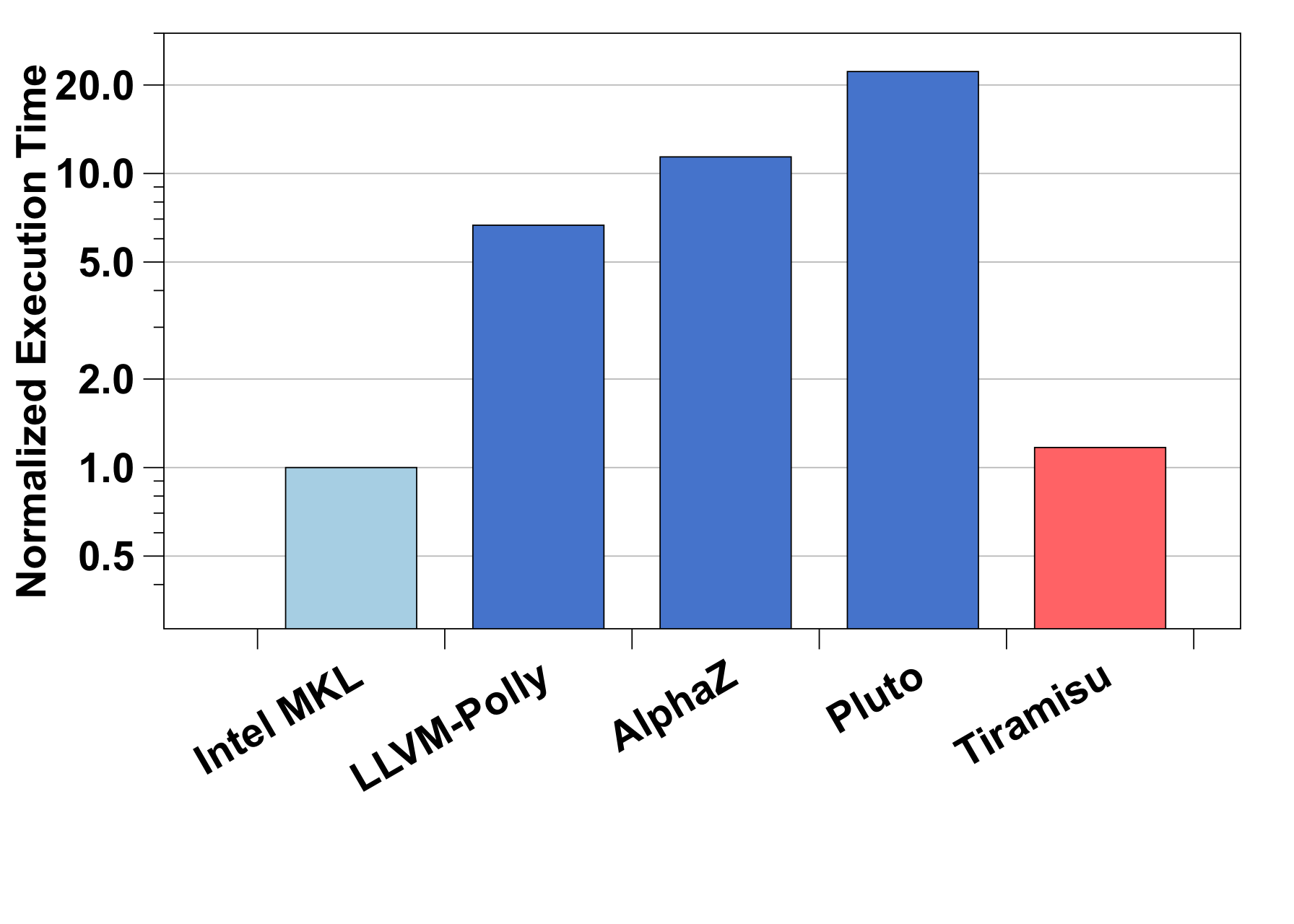GEMM - Comparison with Polyhedral Compilers (CPU)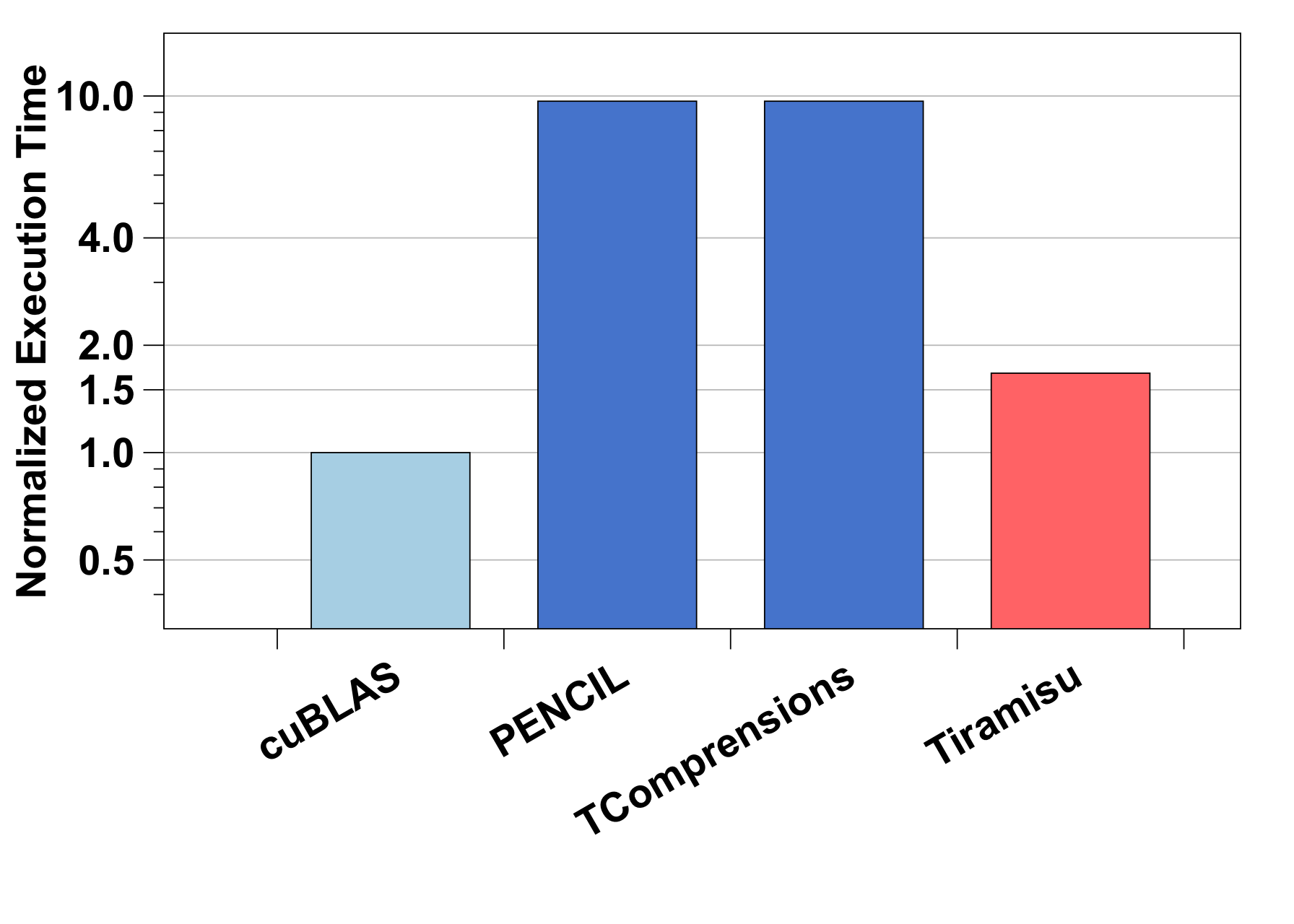GEMM - Comparison with Polyhedral Compilers (GPU)

Matrix dimension sizes for CPU: 1060x1060x1060. GPU: 3072x3072x3072.